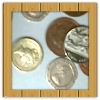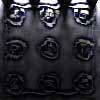#### You may also like### Win or Lose?

A gambler bets half the money in his pocket on the toss of a coin, winning an equal amount for a head and losing his money if the result is a tail. After 2n plays he has won exactly n times. Has he more money than he started with?### Scratch Cards

To win on a scratch card you have to uncover three numbers that add up to more than fifteen. What is the probability of winning a prize?### In a Box

Chris and Jo put two red and four blue ribbons in a box. They each pick a ribbon from the box without looking. Jo wins if the two ribbons are the same colour. Is the game fair?

# Fixing the Odds

##### Age 14 to 16Challenge Level

Again we had the solution to this question from Joel, well done Joel.

1. You have two bags, four red balls and four white balls. You must put all the balls in the bags although you are allowed to have one bag empty. Your friend chooses a bag at random and then chooses a ball at random from that bag. How should you distribute the balls between the two bags so as to make the probability that your friend will choose a red ball as small as possible and what will the probability be in that case?

The solution is to put all the balls into one bag, then (for any number of balls, say n red and n white)

P(red) = 1/2 (1/2) + 1/2 (0) = 1/4.

2. How should you distribute the balls so as to make the probability of choosing a red ball as large as possible and what will the probability be in that case?

The solution here is to put no white balls and at least one red in one bag, then the probability of choosing that bag and a red from it is 1/2, which is maximal. In order to make the probability of choosing a red from the other bag as large as possible we put as many reds in that bag as possible, which means putting exactly one red and no whites in the first bag and all the remaining balls in the second bag. With 4 red and 4 white balls the probability of selecting a red ball is then 5/7.

3. What happens if you have two bags, a hundred red balls and a hundred white balls?

The case for n = 100 is left to the reader. In general, with 1 red in one bag and all the remaining balls in the other bag, the probability of drawing a red ball tends to a limit as n tends to infinity. You may like to find this limiting value.